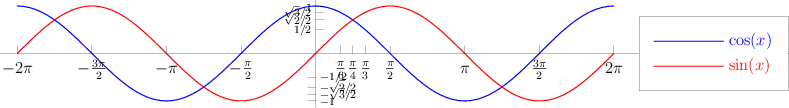# Sinusoid

(diff) ← Older revision | Latest revision (diff) | Newer revision → (diff)
Jump to: navigation, search

The graph of the function $y=\sin x$ (see Fig.). The sinusoid is a continuous curve with period $T=2\pi$. It intersects the $x$-axis at the points $(k\pi, 0)$. These are also points of inflection, meeting the $x$-axis at an angle of $\pm\pi/4$. The extrema are at the points $((k+1/2)\pi, (-1)^k)$.The graph of $y=\cos x = \sin(x+\pi/2)$ is the cosinusoid, obtained by shifting the sinusoid a distance of $\pi/2$ to the left. The cosinusoid intersects the $x$-axis at the points $((k+1/2)\pi,0)$, and its extrema are at the points $(k\pi, (-1)^k)$.

Many oscillatory processes can be described by a periodic function of the form y=a\sin(bx+c), where $a$, $b$ and $c$ are constants and $b>0$. The graph of this function (called a general sinusoid) is obtained from the graph of $y=\sin x$ (the ordinary sinusoid) as follows: expand in the direction of the $y$-axis by a factor $|a|$, contract in the $x$-direction by a factor $b$, translate to the left over a distance $c/b$, and when $a<0$: reflect in the $x$-axis. Its period is $T=2\pi/b$ and it meets the $x$-axis at the points $((k\pi-c)/b,0)$. Its extrema are at the points $(((k+1/2)\pi-c)/b,(-1)^ka)$.

In this article, $k\in \mathbb Z$.

See also Sine; Trigonometric functions.

How to Cite This Entry:
Sinusoid. Encyclopedia of Mathematics. URL: http://www.encyclopediaofmath.org/index.php?title=Sinusoid&oldid=35602
This article was adapted from an original article by Yu.A. Gor'kov (originator), which appeared in Encyclopedia of Mathematics - ISBN 1402006098. See original article